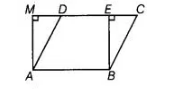# In figure, if parallelogram`
Question:

In figure, if parallelogram $\mathrm{ABCD}$ and rectangle $\mathrm{ABEM}$ are of equal area, then

(a) perimeter of ABCD = perimeter of ABEM

(b) perimeter of ABCD < perimeter of ABEM

(c) perimeter of ABCD > perimeter of ABEM

(d) perimeter of ABCD = ½ (perimeter of ABEM)Solution:

(c) In rectangle ABEM, AB = EM [sides of rectangle] and in parallelogram ABCD, CD = AB

On adding, both equations, we get

AB + CD = EM + AB …(i)

We know that, the perpendicular distance between two parallel sides of a parallelogram is always less than the length of the other parallel sides.

BE < BC and AM < AD

[since, in a right angled triangle, the hypotenuse is greater than the other side]

On adding both above inequalities, we get

SE + AM BE + AM

On adding AB + CD both sides, we get

AB + CD + BC + AD> AB + CD + BE + AM

=> AB+BC + CD + AD> AB+BE + EM+ AM    [∴ CD = AB = EM]

Perimeter of parallelogram ABCD > perimeter of rectangle ABEM